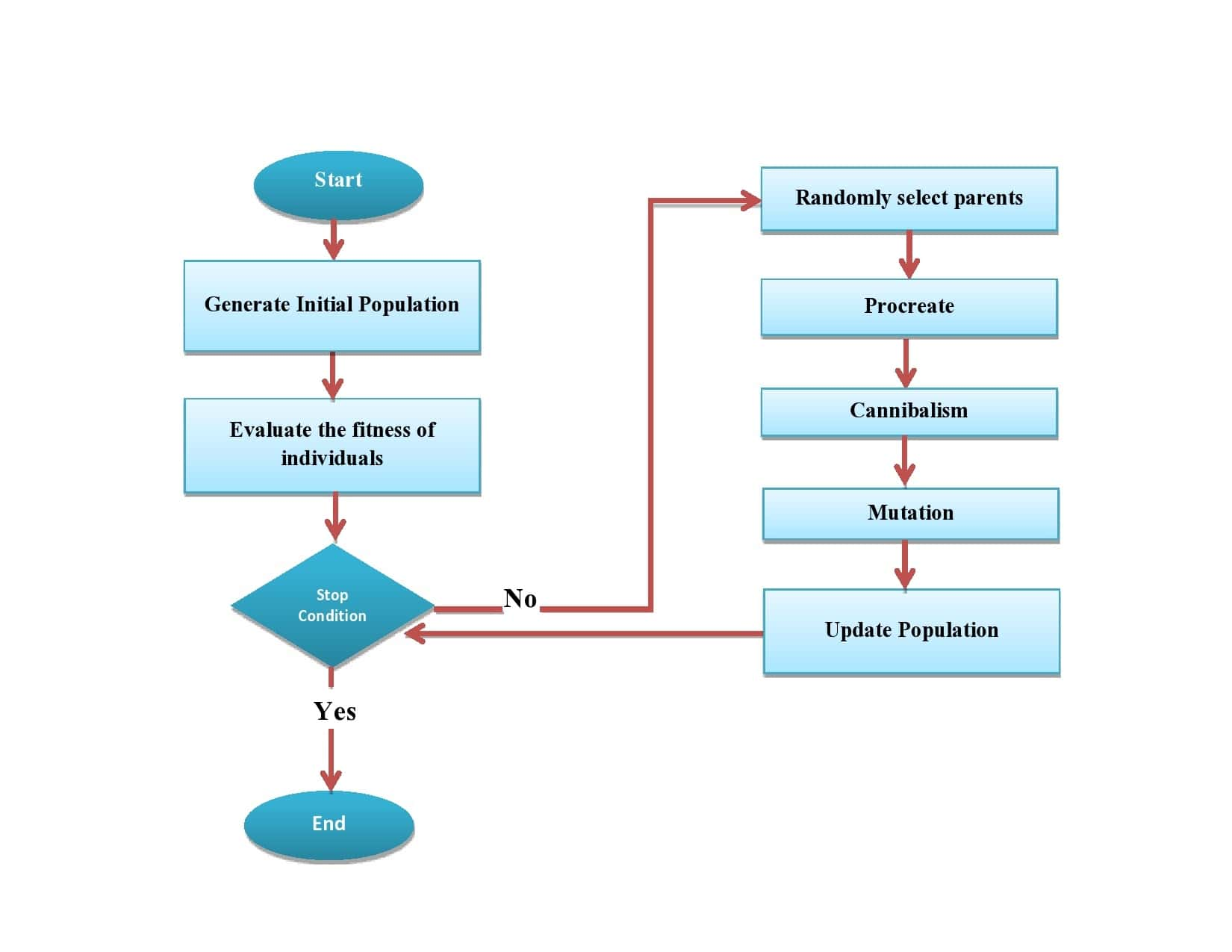## 1. Introduction

Because of their simplicity and versatility, we use metaheuristic algorithms to solve a wide range of engineering and scientific challenges. In particular, they have emerged as effective methods for solving NP problems exactly or approximately.

In this tutorial, we’ll go over a recently proposed metaheuristic algorithm: Black Widow Optimization (BWO).

## 2. Inspiration From Nature

The inspiration for the BWO algorithm came from the mating process of black widow spiders.

Firstly, the black widow spider cannibalizes its mate after mating. Secondly, the spider displays aggressive behavior to capture prey. Furthermore, the spider builds its agents in a specific pattern to maximize its chances of catching prey.

The algorithm mirrors those behavioral patterns by destroying weak spiders (solutions) and guiding the population of artificial agents to the optimal solution following an artificial spider agent.

## 3. Black Widow Optimization (BWO)

In this section, we will go deeper into the mechanism of BWO.

### 3.1. Overview

Here is the flowchart of the algorithm:BWO starts by randomly initializing the population of agents referred to as the widows. Using a specially designed score, these agents are then evaluated based on their fitness for the problem at hand.

Subsequently, the algorithm pairs up the best agents and eliminates the weakest through a process of mating and cannibalism. The position of these agents in the solution space is then used to construct a web that facilitates the search for the best solution.

As the algorithm progresses, the group of agents (population) is continuously updated to improve its overall fitness. The process continues until a satisfactory solution, or a predetermined stopping point is reached. This means that the solutions gradually become better and better (based on the fitness value returned from the objective/fitness function), aiming to find a single global optimal solution (the best solution compared to all others in the population).

The combination of mating, cannibalism, and web building helps the Black Widow Optimization algorithm to navigate complex optimization problems and find optimal solutions efficiently.

## 4. Pseudocode

Here’s the pseudocode:First, we set the algorithm’s parameters: the number of widows and the reproduction, cannibalism, and mutation rates. Additionally, we define a fitness function to evaluate the widows, i.e., candidate solutions.

The algorithm starts by randomly initializing the population of widows. Then, it iteratively applies the procreation (reproduction), cannibalism, and mutation steps.

### 4.1. Procreation

In the procreation phase, we randomly select widows for mating. As a result, we get their offspring, a set of solutions derived from the parents.

Letbe the problem’s dimensionality andandbe two individuals (-dimensional vectors) selected for mating. We getchildren by running the following two stepstimes:

[details]Here,is a-dimensional array of random numbers from, andandrepresent the offspring.

### 4.2. Cannibalism

Cannibalism is when spiders eat members of the same species. In nature, there are three scenarios of cannibalism: when a female eats its mate, when babies eat their mother, or when a strong baby eats its sibling.

BWO algorithm does cannibalism in two ways.

First, it destroys the father after each mating. When two widows mate, the father is the solution with a worse fitness value.

Another type of cannibalism is called sibling cannibalism. We implement it by keeping several top solutions we get as children in the reproduction step.

### 4.3. Mutation

In the mutation phase, we select a random number of individuals from the children population. Each of the chosen solutions randomly exchanges its two elements.

After applying mutation, we replace the old population of spiders with the.

### 4.4. Stopping Criteria and the Population Size

The algorithm goes on until meeting the stopping criteria. For example, until reaching the maximum number of iterations.

To keep the population size constant atthroughout the algorithm, the number of children that survive an iteration must be equal to the number of widows removed from the population.

Thus, the number of parents selected for procreation is, half of which are fathers, so the number of spiders removed in an iteration is. The total number of children that survive an iteration is:To maintain a population size of, that number should be equal to, i.e.:## 5. Conclusion

In this article, we talked about the Black Widow Optimization algorithm (BWO). The inspiration for the BWO came from the unique mating behavior of the black widow spiders in nature.

The Black Widow Optimization algorithm has been tested on different problems and works well in terms of finding solutions quickly and accurately. It has also been compared to other popular optimization algorithms and does just as well as them.